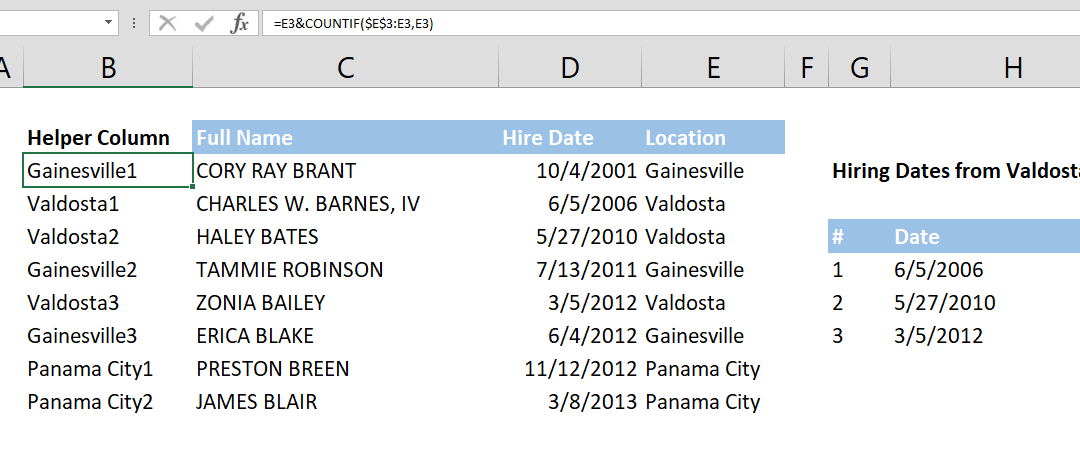## 10 Things You Need to Know About the VLOOKUP Excel Function

In this guide we’re going to go over 10 things you should know about the frequently-used-yet-not-so-easy-to-use VLOOKUP Excel function. Although this guide mainly focuses on the VLOOKUP function, these tips also apply to the HLOOKUP function, which essentially...## How to perform reverse lookup with CHOOSE function

Lookup functions are widely used in Excel models. However, one of the most popular lookup functions, VLOOKUP, can only search the left-most column in the search area. This function will fall short if you need to search in the middle of a table and return values from...

## How to speed up lookup formulas

Lookup functions are extremely useful when working with large data sets. Although they almost always work instantaneously with small data, larger data sets is when things can get rough in terms of performance, as large operations can require more computational...## How to convert Excel columns to rows - Unpivoting

This article explains how to convert Excel columns to rows, also knows us unpivoting, using VLOOKUP formula. Unpivoting is the opposite of pivoting data (see Pivot Tables) by distributing values from a single column to multiple columns while keeping unique values in...## How to lookup across two tables with VLOOKUP and IF functions

How to lookup across two tables by a condition is a combination of the VLOOKUP and the IF functions. The IF function handles which table and VLOOKUP handles what to search for. Syntax =VLOOKUP(lookup value, IF(condition, first table if condition is TRUE, second table...## How to calculate Basic Tax Rate with VLOOKUP

The tax brackets can be confusing to work with. Different ‘levels’ of your income needs to be multiplied with a different percentage to find the total. This can be done with the conventional Excel formulas like IF and a bunch of others, but that’s the long and hard to...## How to get nth match with VLOOKUP

This article shows you how to get the nth match with VLOOKUP formula. Unfortunately, Excel doesn’t have a built-in function to find any value beyond the first match. Syntax Unique lookup value: =lookup value & COUNTIF(expanding range of lookup values, lookup...